# EDS Case Study¶

Explore changes in political views

Allen Downey

In :
# If we're running in Colab, set up the environment

import sys

if IN_COLAB:
!pip install empiricaldist
!git clone --depth 1 https://github.com/AllenDowney/ExploratoryDataAnalysis
%cd ExploratoryDataAnalysis

In :
import pandas as pd
import numpy as np
import matplotlib.pyplot as plt
import seaborn as sns

from utils import decorate


In :
gss = pd.read_hdf('eds.gss.hdf5', 'gss0')
gss.shape

Out:
(64814, 105)

### Validating polviews¶

polviews contains responses to the following question

We hear a lot of talk these days about liberals and conservatives. I'm going to show you a seven-point scale on which the political views that people might hold are arranged from extremely liberal--point 1--to extremely conservative--point 7. Where would you place yourself on this scale?

values takes a variable column and returns a series of values and their frequencies.

In :
def values(series):
"""Count the values and sort.

series: pd.Series

returns: series mapping from values to frequencies
"""
return series.value_counts().sort_index()


Here's the distribution of values for polviews

In :
values(gss['polviews'])

Out:
1.0     1699
2.0     6299
3.0     6981
4.0    21444
5.0     8864
6.0     8495
7.0     1770
Name: polviews, dtype: int64

If we select only records from 1974, we can compare the values to the table in the codebook.

In :
gss74 = gss['year']==1974
gss74.sum()

Out:
1484

The following values are consistent with the codebook, which confirms that we are reading the right variable and interpreting it correctly.

In :
polviews74 = gss.loc[gss74, 'polviews']
values(polviews74)

Out:
1.0     31
2.0    201
3.0    211
4.0    538
5.0    223
6.0    181
7.0     30
Name: polviews, dtype: int64

Let's compare the results from 1974 and 2018.

In :
gss18 = gss['year'] == 2018
polviews18 = gss.loc[gss18, 'polviews']


Here are the two distributions plotted side by side. I use the Pmf class from empiricaldist to compute the probability mass functions (PMFs).

In :
from empiricaldist import Pmf

Pmf.from_seq(polviews74).bar(label='1974', width=-0.45, align='edge', alpha=0.7)
Pmf.from_seq(polviews18).bar(label='2018', width=0.45, align='edge', alpha=0.7)

decorate(xlabel='Political view on a 7-point scale',
ylabel='Fraction of population',
title='Distribution of political views')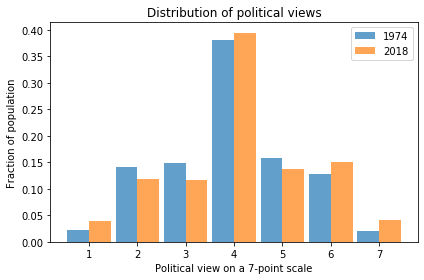### Mean and standard deviation¶

To see how the distribution changes over time, we can group by year and compute the mean of polviews during each year.

In :
by_year = gss.groupby('year')
by_year

Out:
<pandas.core.groupby.generic.DataFrameGroupBy object at 0x7f86e3c3c390>

Here's what the plot looks like.

In :
series = by_year['polviews'].mean()
series.plot(label='polviews')
decorate(ylabel='Mean (7 point scale)',
title='Mean of polviews')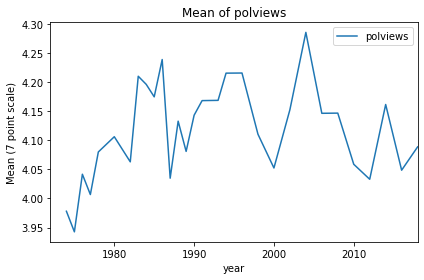The standard deviation quantifies the spread of the distribution, which is one way to measure polarization.

In :
series = by_year['polviews'].std()
series.plot(color='C1', label='polviews')
decorate(ylabel='Standard deviation (7 point scale)',
title='Standard deviation of polviews')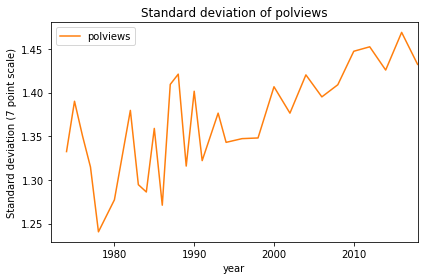Both plots are quite noisy.

### Local regression¶

We can use local regression to compute a smooth line through these data points.

In :
from statsmodels.nonparametric.smoothers_lowess import lowess

def make_lowess(series):
"""Use LOWESS to compute a smooth line.

series: pd.Series

returns: pd.Series
"""
endog = series.values
exog = series.index.values

smooth = lowess(endog, exog)
index, data = np.transpose(smooth)

return pd.Series(data, index=index)


We'll use the following function to plot data points and the smoothed line.

In :
def plot_series_lowess(series, color):
"""Plots a series of data points and a smooth line.

series: pd.Series
color: string or tuple
"""
series.plot(lw=0, marker='o', color=color, alpha=0.5)
smooth = make_lowess(series)
smooth.plot(label='_', color=color)


colors is a dictionary that maps from column name to color, using conventional colors for conservatives and liberals.

In :
palette = sns.color_palette('muted', 5)
sns.palplot(palette)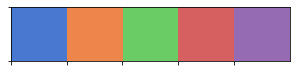In :
colors = {'Conservative': palette,
'Moderate': palette,
'Liberal': palette}


The following figure shows the mean of polviews and a smooth line.

In :
series = by_year['polviews'].mean()
plot_series_lowess(series, 'C2')
decorate(ylabel='Mean (7 point scale)',
title='Mean of polviews',
xlabel='Year',
xlim=[1972, 2020])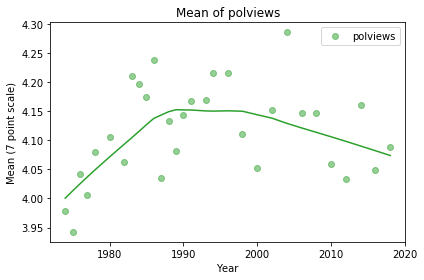And here's the standard deviation of polviews with a smooth line.

In :
series = by_year['polviews'].std()
plot_series_lowess(series, color='C4')
decorate(ylabel='Standard deviation (7 point scale)',
title='Standard deviation of polviews',
xlabel='Year',
xlim=[1972, 2020])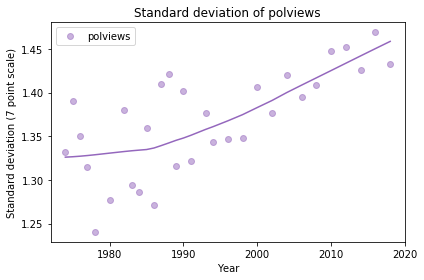### Categorical data¶

In the previous sections, we treated polviews as a numeric quantity, but it's really categorical. So let's see what we can do with it as a categorical variable.

In :
# recode the 7 point scale with words
d = {1:'Extremely liberal',
2:'Liberal',
3:'Slightly liberal',
4:'Moderate',
5:'Slightly conservative',
6:'Conservative',
7:'Extremely conservative'}

gss['polviews7'] = gss.polviews.replace(d)
values(gss['polviews7'])

Out:
Conservative               8495
Extremely conservative     1770
Extremely liberal          1699
Liberal                    6299
Moderate                  21444
Slightly conservative      8864
Slightly liberal           6981
Name: polviews7, dtype: int64

The following function computes a DataFrame with the number of respondents in each category for each year.

In :
def count_by_year(gss, varname):
"""Groups by category and year and counts.

gss: DataFrame
varname: string variable to group by

returns: DataFrame with one row per year, one column per category.
"""
grouped = gss.groupby([varname, 'year'])
count = grouped[varname].count().unstack(level=0)

# note: the following is not ideal, because it does not
# distinguish 0 from NA, but in this dataset the only
# zeros are during years when the question was not asked.
count = count.replace(0, np.nan).dropna()
return count


Here are the first few lines from the result. We can compare the rows in this table with the columns in the codebook, confirming that the computation is correct, so far.

In :
polviews7_count = count_by_year(gss, 'polviews7')

Out:
polviews7 Conservative Extremely conservative Extremely liberal Liberal Moderate Slightly conservative Slightly liberal
year
1974 181 30 31 201 538 223 211
1975 162 45 56 184 540 204 207
1976 206 34 31 198 564 209 175
1977 164 42 37 181 594 243 214
1978 187 25 21 140 559 265 255

The number of respondents varies from year to year, so we need to "normalize", computing the fraction of respondents in each category, each year.

The following function computes the total in each row and divides through, so each row adds up to 1.

In :
def normalize_by_year(df):
"""Divide each row by its total.

df: DataFrame with one year per row, one category per column
"""
# add up the elements of each row
total = df.sum(axis=1)

# divide each column by the total
return df.div(total, axis=0)


Here's what that looking like for the 7-point scale.

In :
polviews7_norm = normalize_by_year(polviews7_count)

Out:
polviews7 Conservative Extremely conservative Extremely liberal Liberal Moderate Slightly conservative Slightly liberal
year
1974 0.127915 0.021201 0.021908 0.142049 0.380212 0.157597 0.149117
1975 0.115880 0.032189 0.040057 0.131617 0.386266 0.145923 0.148069
1976 0.145378 0.023994 0.021877 0.139732 0.398024 0.147495 0.123500
1977 0.111186 0.028475 0.025085 0.122712 0.402712 0.164746 0.145085
1978 0.128788 0.017218 0.014463 0.096419 0.384986 0.182507 0.175620

The following function takes a DataFrame and plots the columns using plot_series_lowess.

In :
def plot_columns_lowess(df, columns, colors):
"""Plot the columns in a DataFrame.

df: pd.DataFrame
columns: list of column names, in the desired order
colors: mapping from column names to colors
"""
for col in columns:
series = df[col]
plot_series_lowess(series, colors[col])


We can get the column names from the dictionary we used to recode.

In :
columns = d.values()
columns

Out:
dict_values(['Extremely liberal', 'Liberal', 'Slightly liberal', 'Moderate', 'Slightly conservative', 'Conservative', 'Extremely conservative'])

I'll use purple for moderates and a divergent palette from blue to red for the other categories.

In :
muted = sns.color_palette('muted', 5)
purple = muted

In :
palette = sns.color_palette('RdBu_r', 7)
palette = purple
sns.palplot(palette)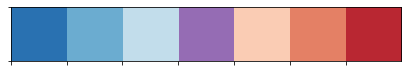Here's the color dictionary.

In :
colors = dict(zip(columns, palette))


The following function sets the position of the figure legend.

In :
def anchor_legend(x, y):
plt.legend(bbox_to_anchor=(x, y), loc='upper left', ncol=1)


Here are the 7 categories plotted as a function of time.

In :
plot_columns_lowess(polviews7_norm, columns, colors)
decorate(xlabel='Year',
ylabel='Proportion',
title='Fraction of people with each political view',
xlim=[1970, 2020])

anchor_legend(1.02, 1.02)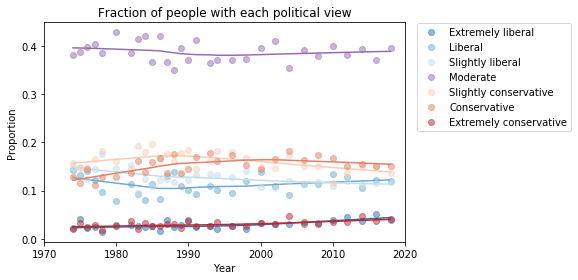Here's a close look at the extremes.

In :
columns2 = ['Extremely liberal', 'Extremely conservative']

plot_columns_lowess(polviews7_norm, columns2, colors)
decorate(xlabel='Year',
ylabel='Proportion',
title='Fraction of people with each political view',
xlim=[1970, 2020])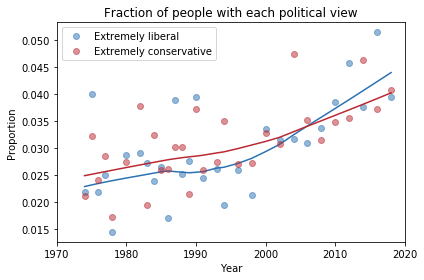### 3-point scale¶

To make it easier to visualize groups, I'm going to lump the 7-point scale into a 3-point scale.

With this scale, there are roughly the same number of people in each group.

In :
# replace 7 point scale with 3 point scale
d = {1:'Liberal (all)',
2:'Liberal (all)',
3:'Liberal (all)',
4:'Moderate',
5:'Conservative (all)',
6:'Conservative (all)',
7:'Conservative (all)'}

gss['polviews3'] = gss.polviews.replace(d)
values(gss['polviews3'])

Out:
Conservative (all)    19129
Liberal (all)         14979
Moderate              21444
Name: polviews3, dtype: int64

Here's the number of people in each category, over time.

In :
polviews3_count = count_by_year(gss, 'polviews3')

Out:
polviews3 Conservative (all) Liberal (all) Moderate
year
1974 434 443 538
1975 411 447 540
1976 449 404 564
1977 449 432 594
1978 477 416 559

And here are the normalize counts.

In :
polviews3_norm = normalize_by_year(polviews3_count)

Out:
polviews3 Conservative (all) Liberal (all) Moderate
year
1974 0.306714 0.313074 0.380212
1975 0.293991 0.319742 0.386266
1976 0.316867 0.285109 0.398024
1977 0.304407 0.292881 0.402712
1978 0.328512 0.286501 0.384986
In :
polviews3_norm.tail()

Out:
polviews3 Conservative (all) Liberal (all) Moderate
year
2010 0.317468 0.284051 0.398481
2012 0.324109 0.294305 0.381586
2014 0.351351 0.255119 0.393530
2016 0.339376 0.290428 0.370196
2018 0.329495 0.275908 0.394597

Here's the color dictionary.

In :
colors = {'Conservative (all)': muted,
'Moderate': muted,
'Liberal (all)': muted}


The following plot shows the fraction of respondents in each category over time.

In :
columns = ['Moderate', 'Conservative (all)', 'Liberal (all)']
plot_columns_lowess(polviews3_norm, columns, colors)
decorate(xlabel='Year',
ylabel='Proportion',
title='Fraction of people with each political view',
xlim=[1970, 2020])

anchor_legend(1.02, 1.02)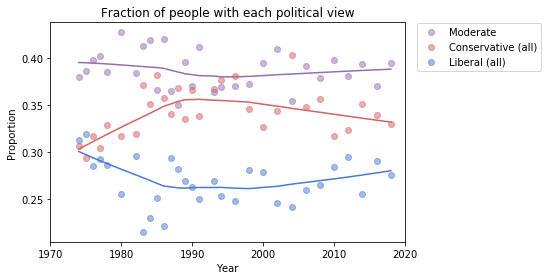In [ ]:


In [ ]: